## REPEAT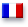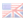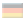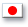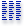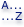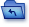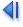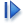version 11

REPEAT (arithmetic_expression, arithmetic_expression)

Description

The REPEAT function returns the first arithmetic_expression repeated the requested number of times (passed in second arithmetic_expression).

Example

This example illustrates how it works:

```   SELECT REPEAT( 'repeat', 3 )
` returns 'repeatrepeatrepeat'
```